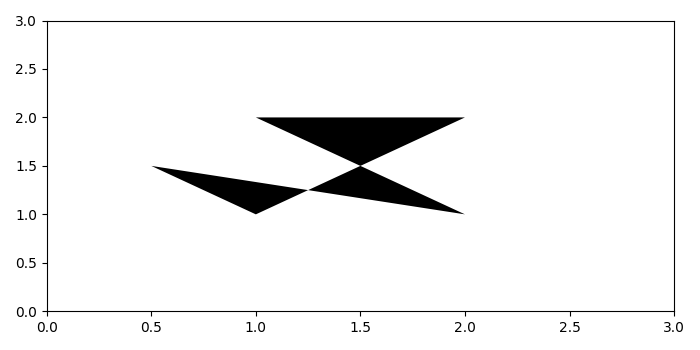# How to draw the largest polygon from a set of points in matplotlib?

To draw the largest polygon from a set of points in matplotlib, we can take the following steps −

• Import "Polygon" from matplotlib.patches.
• Set the figure size and adjust the padding between and around the subplots.
• Create a list of data points for the largest polygon.
• Get the polygon instance.
• Create a figure and a set of subplots.
• Add a polygon instance patch.
• Set the x and y scale limit.
• To display the figure, use show() method.

## Example

import numpy as np
import matplotlib.pyplot as plt
from matplotlib.patches import Polygon

plt.rcParams["figure.figsize"] = [7.00, 3.50]
plt.rcParams["figure.autolayout"] = True

y = np.array([[1, 1], [0.5, 1.5], [2, 1], [1, 2], [2, 2]])

p = Polygon(y, facecolor='k')

fig, ax = plt.subplots()

ax.set_xlim([0, 3])
ax.set_ylim([0, 3])

plt.show()

## Output

It will produce the following outputUpdated on: 20-Sep-2021

295 Views